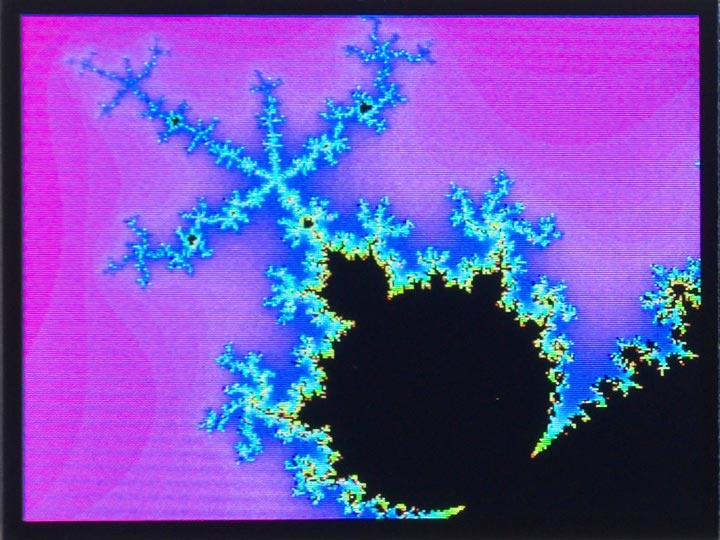## Mandelbrot set

This popular fractal function generates complex detail however far you zoom in to it .For each point (x, y) the function repeatedly applies a transformation to the complex number represented by x + iy, and counts the number of iterations before the size of the value exceeds 2.

The number of iterations is plotted as a different colour, using the hsv routine in Graphics utilities, or black if the function hasn't diverged after 80 iterations:

```(defun mandelbrot (x0 y0 scale)
(set-rotation 2)
(fill-screen)
(dotimes (y 240)
(let ((b0 (+ (/ (- y 120) 120 scale) y0)))
(dotimes (x 320)
(let* ((a0 (+ (/ (- x 160) 120 scale) x0))
(c 80) (a a0) (b b0) a2)
(loop
(setq a2 (+ (- (* a a) (* b b)) a0))
(setq b (+ (* 2 a b) b0))
(setq a a2)
(decf c)
(when (or (> (+ (* a a) (* b b)) 4) (zerop c)) (return)))
(draw-pixel x y (if (plusp c) (hsv (* 359 (/ c 80)) 1 1) 0)))))))```

The parameters x0 and y0 are the coordinates of the centre of the plot, and scale specifies the scale factor. To plot the whole Mandelbrot set call:

`(mandelbrot -0.5 0 1)`

The plot shown above zooms in on a detail, and was obtained with:

`(mandelbrot -0.53 -0.61 11)`

On a MAiX board at the default clock speed it took 127 s to plot the above detail on a 320 x 240 display.

For a description of how to speed this up by writing the inner loop in RISC-V assembler see Mandelbrot set using assembler.

1. ^ Mandelbrot set on Wikipedia.

Previous: Dragon curve

Next: Barnsley Fern